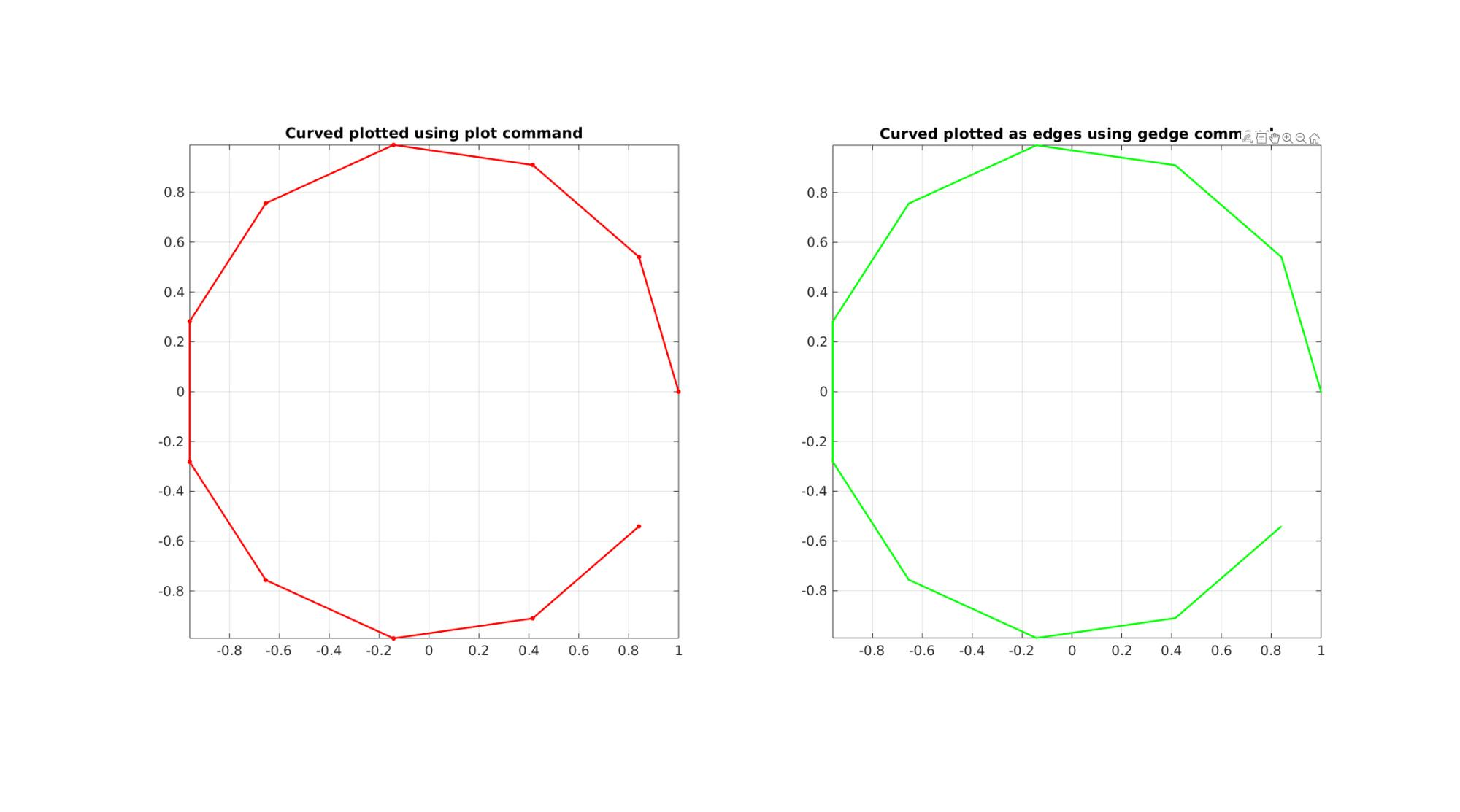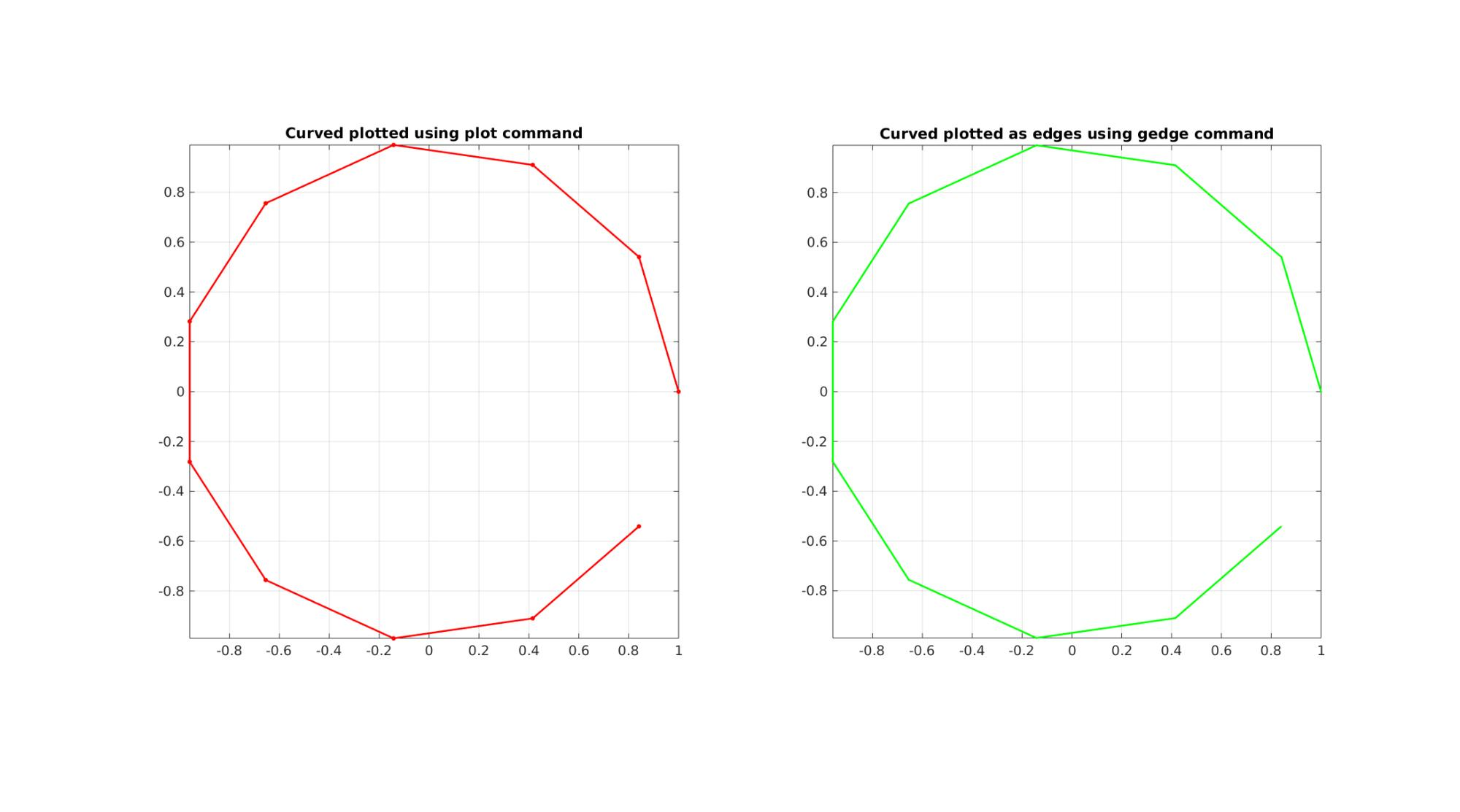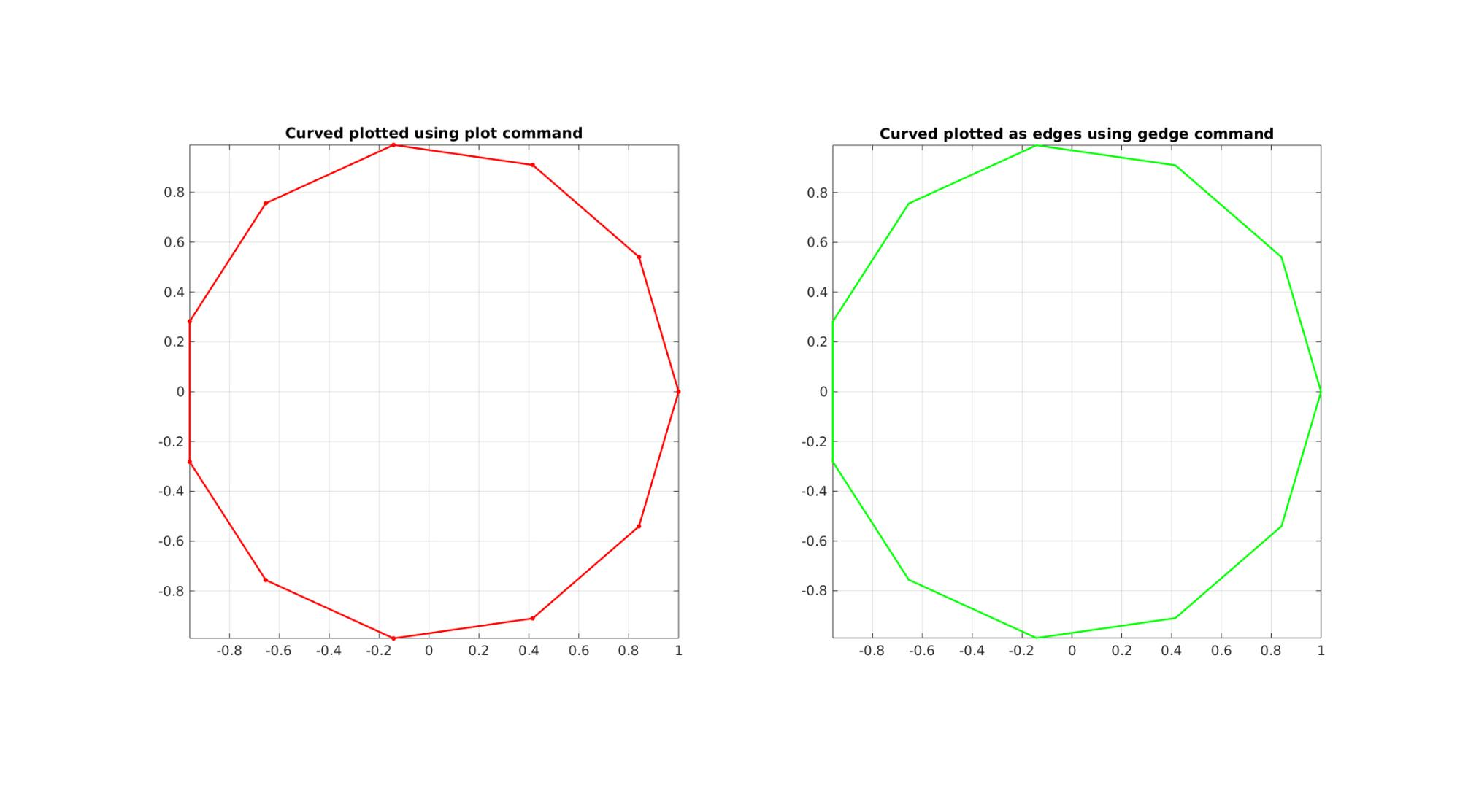# curveToEdgeList

Below is a demonstration of the features of the curveToEdgeList function

## Contents

```clear; close all; clc;
```

## Syntax

[E]=curveToEdgeList(N);

## Description

This function creates an edge array E for the input curve defined by N. The input parameter N can be of the following type: * A single scalar In this case it is assumed N defines the number of points on the curve * An nxm array representing n points and m dimensions, in this case it is assumed N represents the vertex array for the curve * A row or column array, in this case it is assumed that N defines the indices for the points defining the curve.

If closeCurveOpt=1 it is assumed the start and end of the curve should be attached.

## Examples

Plot settings

```lineWidth=2;
markerSize=15;
fontSize=15;
```

## Example 1: Creating an edge array for a curve

Create example curve data

```t=linspace(0,2*pi,12)';
t=t(1:end-1);
V=[cos(t) sin(t) zeros(size(t))]; %Curve vertices
n=size(V,1); %Number of points in curve
ind=1:1:n; %Curve point indices
```

Get curve edge array

```% Use vertices
[E]=curveToEdgeList(V)

% Use number of points
[E]=curveToEdgeList(n)

% Use curve indices
[E]=curveToEdgeList(ind)
```
```E =

1     2
2     3
3     4
4     5
5     6
6     7
7     8
8     9
9    10
10    11

E =

1     2
2     3
3     4
4     5
5     6
6     7
7     8
8     9
9    10
10    11

E =

1     2
2     3
3     4
4     5
5     6
6     7
7     8
8     9
9    10
10    11

```

Visualization

```cFigure;
subplot(1,2,1); hold on;
title('Curved plotted using plot command');
plotV(V,'r.-','LineWidth',lineWidth,'MarkerSize',markerSize);
axis tight; axis equal; grid on; box on; view(2);
set(gca,'FontSize',fontSize);
subplot(1,2,2); hold on;
title('Curved plotted as edges using gedge command');
gedge(E,V,'g',2);
axis tight; axis equal; grid on; box on; view(2);
set(gca,'FontSize',fontSize);
drawnow;
```## Example 2: Using closeCurveOpt

Use closeCurveOpt

```closeCurveOpt=1;
[E]=curveToEdgeList(ind,closeCurveOpt)
```
```E =

1     2
2     3
3     4
4     5
5     6
6     7
7     8
8     9
9    10
10    11
11     1

```

Visualization

```cFigure;
subplot(1,2,1); hold on;
title('Curved plotted using plot command');
plotV(V([1:end 1],:),'r.-','LineWidth',lineWidth,'MarkerSize',markerSize);
axis tight; axis equal; grid on; box on; view(2);
set(gca,'FontSize',fontSize);
subplot(1,2,2); hold on;
title('Curved plotted as edges using gedge command');
gedge(E,V,'g',2);
axis tight; axis equal; grid on; box on; view(2);
set(gca,'FontSize',fontSize);
drawnow;
```GIBBON www.gibboncode.org

Kevin Mattheus Moerman, [email protected]

GIBBON footer text

GIBBON: The Geometry and Image-based Bioengineering add-On. A toolbox for image segmentation, image-based modeling, meshing, and finite element analysis.

Copyright (C) 2006-2023 Kevin Mattheus Moerman and the GIBBON contributors

This program is free software: you can redistribute it and/or modify it under the terms of the GNU General Public License as published by the Free Software Foundation, either version 3 of the License, or (at your option) any later version.

This program is distributed in the hope that it will be useful, but WITHOUT ANY WARRANTY; without even the implied warranty of MERCHANTABILITY or FITNESS FOR A PARTICULAR PURPOSE. See the GNU General Public License for more details.

You should have received a copy of the GNU General Public License along with this program. If not, see http://www.gnu.org/licenses/.